自编码器_自编码器实现 - CSDN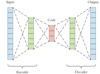autoencoder, AE

1987年 

Yann LeCun 

• 自编码器的原理及实现 1. 什么是自编码器 自编码器就是将原始数据进行编码，进行降低维度，发现数据之间的规律的过程。 数据降维比如mnist的图片为28*28像素，将图片向量化之后的得到一个长度为784的向量。在网络...
自编码器的原理及实现
1. 什么是自编码器
自编码器就是将原始数据进行编码，进行降低维度，发现数据之间的规律的过程。
数据降维比如mnist的图片为28*28像素，将图片向量化之后的得到一个长度为784的向量。在网络训练的过程中，网络只用到该向量之中少量元素，其中的大部分元素对于网络来说是没有用的，自编码器通过无监督学习来提取有用的信息，对于手写数字图片来说可能是颜色为黑色的像素点，将图片中的很大一部分白色像素舍弃，只提取对网络有用的信息，到达降低数据维度的目的。
作用

先对无标注的数据进行自编码器的训练，然后将有标注的数据第一步输入自编码器，自编码器的输出输入到神经网络之中，达到提升网络输出精度的目的。

用于神经网络权重的初始化。

基本形式如下，其中f(x)为编码函数，g(x)为解码函数
$x\overset{f(x)}{\rightarrow}h\overset{g(x)}{\rightarrow}x'$
训练的过程，我们采用下面形式的约束
$x\approx x'$
即设计一个损失函数，让编码器的输入和输出尽可能相似
其中
$x'=g(f(x))$
但自编码器学习的目的不是学习上面这个恒等函数，这可能导致过拟合，我们要自编码器学习的是将稀疏数据到稠密的一个映射，而不是输入和输出完全相等，这样提取的特征才能为后面的网络所用。
损失函数的设计
以mnist数据集为例，设输入x的数据是长度为784的一维向量，编码后h是长度为196的一维向量，解码后x’是长度为784的一维向量。
均方误差（MSE） 用来衡量输入数据和输出数据的相似度。表示如下
$L(x,g(f(x))=E_{x\sim~p_{data}}\left\|x-g(f(x))\right\|^2$
在学习的过程中，均方误差可能变得很小，这样会导过拟合，而我们期望的是一个泛化能力很强的编码器，所以我们加如L1正则化和相对熵(KLD)来抑制过拟合。
L1正则化仅仅作用于编码，因为我们关心的是编码的过程，解码器只是方便显示编码之后的结果。
$\Omega(h)=\lambda\sum\nolimits_{i}\left|h_i\right|$
$h_i$是第$i$个神经元的激励值。
相对熵（KL Divergence，KLD）。首先定义隐层神经元j的平均活跃度${\hat{\rho}}_j$
$\hat{\rho}_j=\frac{1}{N}\sum\nolimits_{i=1}^{N}{h}^{(i)}_j$
对$i$求和表示对训练集（N个样本）的所有输入取均值，j代表第几个隐层神经元，激励值通常在$0\sim1$之间。对稀疏性的约束就是令神经元的平均活跃度接近稀疏性系数$\rho:\hat{\rho}_j\approx\rho$这个系数通常取接近0的值，从而约束隐层神经元的活跃程度，可以把$\rho$理解成某个神经元被激活的概率。
为了实现这个约束，需要在损失函数中添加一个损失项
$\sum\nolimits_{j=1}^{M}KL(\rho\parallel\hat{\rho}_j)=\sum\nolimits_{j=1}^{M}[\rho\cdot\log\frac{p}{\hat{\rho}_j}+(1-\rho)\cdot\log\frac{1-p}{1-\hat{\rho}_j}]$
KLD是一种衡量两个分布之间差异的方法，式中的$\rho$和$\hat{\rho}_j$分别表示期望和实际的隐层神经元的输出两点分布（两点分别代表饱和和睡眠）的均值和期望。
两种损失函数可定义为下面版本：
L1：
$L(x,g(f(x))=E_{x\sim~p_{data}}\left\|x-g(f(x))\right\|^2+\lambda\sum\nolimits_{i}\left|h_i\right|$
KLD：
$L(x,g(f(x))=E_{x\sim~p_{data}}\left\|x-g(f(x))\right\|^2+\beta\sum_{j=1}^{M}[\rho\cdot\log\frac{p}{\hat{\rho}_j}+(1-\rho)\cdot\log\frac{1-p}{1-\hat{\rho}_j}]$
两种损失函数该如何选择

隐层激活函数类型
重构层激活函数类型
MSE
L1
KLD

Sigmoid
Sigmoid
True
False
True

Relu
Softplus
True
True
False

隐层使用Sigmoid时，隐层输出值在(0,1)之间，可用来计算KLD。隐层使用Relu时，隐层的输出值在$[0,+\infty)$，不能使用KLD。
实现代码如下：
# coding:utf-8

import tensorflow as tf
import tensorlayer as tl
from tensorlayer.layers import *
import numpy as np
import matplotlib.pylab as plt

learning_rate = 0.0001
lambda_l2_w = 0.01
n_epochs = 100
batch_size = 128
print_interval = 200

hidden_size = 196
input_size = 784
image_width = 28
model = 'sigmoid'

X_train, y_train, X_val, y_val, X_test, y_test = tl.files.load_mnist_dataset(path='./data/')

x = tf.placeholder(tf.float32,shape=[None,784],name='x')

print('Build Network')
if model=='relu':
net = InputLayer(x, name='input')
net = DenseLayer(net,n_units=hidden_size,act=tf.nn.relu, name='relu1')
encode_img = net.outputs
recon_layer1 = DenseLayer(net,n_units=input_size,act=tf.nn.softplus,name='recon_layer1')

if model=='sigmoid':
net = InputLayer(x, name='input')
net = DenseLayer(net,n_units=hidden_size,act=tf.nn.sigmoid, name='sigmoid1')
encode_img = net.outputs
recon_layer1 = DenseLayer(net,n_units=input_size,act=tf.nn.sigmoid,name='recon_layer1')

y = recon_layer1.outputs
train_params = recon_layer1.all_params[-4:]

mse = tf.reduce_sum(tf.squared_difference(y,x),1)
mse = tf.reduce_mean(mse)

# w1 和 w2 采用L2正则化
L2_w = tf.contrib.layers.l2_regularizer(lambda_l2_w)(train_params)+\
tf.contrib.layers.l2_regularizer(lambda_l2_w)(train_params)

# 稀疏性约束
activation_out = recon_layer1.all_layers[-2]
L1_a = 0.001 * tf.reduce_mean(activation_out)

# 相对熵（KLD）
beta = 0.5
rho = 0.15
p_hat = tf.reduce_mean(activation_out,0)
KLD = beta * tf.reduce_sum(rho * tf.log(tf.divide(rho,p_hat)) +
(1-rho) * tf.log((1-rho)/(tf.subtract(float(1),p_hat))))

# 联合损失函数
if model=='sigmoid':
cost = mse + L2_w + KLD
if model=='relu':
cost = mse + L2_w + L1_a

# 定义优化器
saver = tf.train.Saver()

# 模型训练
total_batch = X_train.shape // batch_size

with tf.Session() as sess:
sess.run(tf.global_variables_initializer())

for epoch in range(n_epochs):
avg_cost = 0
for i in range(total_batch):
batch_x,batch_y =X_train[i*batch_size:(i+1)*batch_size],y_train[i*batch_size:(i+1)*batch_size]
batch_x = np.array(batch_x).astype(np.float32)
batch_cost, _ = sess.run([cost, train_op], feed_dict={x: batch_x})
if not i % print_interval:
print('Minibatch: %03d | Cost:  %.3f' % (i + 1, batch_cost))
print('Epoch:   %03d | AvgCost:  %.3f' % (epoch + 1, avg_cost / i + 1))
saver.save(sess,save_path='./model/3-101.ckpt')

# 恢复参数
n_images=15
fig,axes=plt.subplots(nrows=2,ncols=n_images,sharex=True,sharey=True,figsize=(20,2.5))

test_images = X_test[:n_images]

with tf.Session() as sess:
# 加载训练好的模型
saver.restore(sess,save_path='./model/3-101.ckpt')

# 获取重构参数
decoded = sess.run(recon_layer1.outputs,feed_dict={x:test_images})
# 恢复编码器的权重参数
if model=='relu':
weights = sess.run(tl.layers.get_variables_with_name('relu1/W:0',False,True))
if model=='sigmoid':
weights = sess.run(tl.layers.get_variables_with_name('sigmoid1/W:0',False,True))

# 获取解码器的权重参数
recon_weights = sess.run(tl.layers.get_variables_with_name('recon_layer1/W:0',False,True))
recon_bias = sess.run(tl.layers.get_variables_with_name('recon_layer1/b:0',False,True))
for i in range(n_images):
for ax,img in zip(axes,[test_images,decoded]):
ax[i].imshow(img[i].reshape(image_width,image_width),cmap='binary')
plt.show()

实验结果:
MSE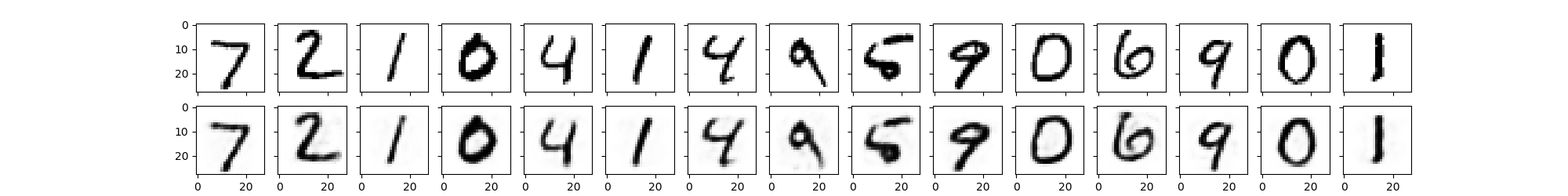MSE+L2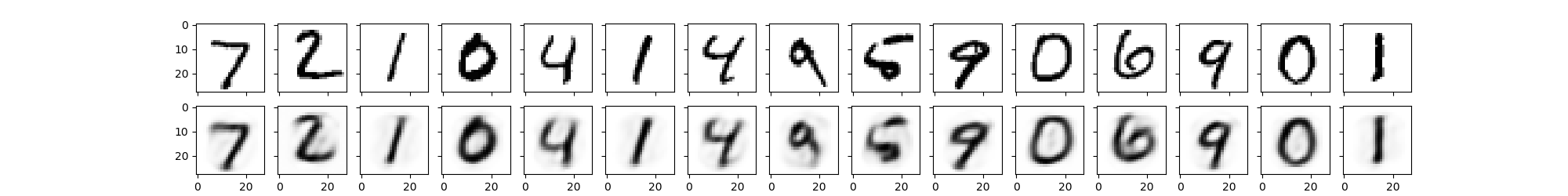MSE+L2+KLD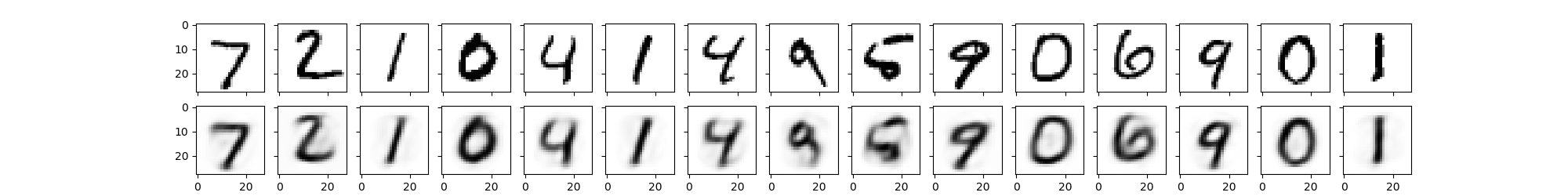总结：
自编码器通过监督学习来发现数据集内部特征，提取有用信息，达到降维的目的。在现实中存在大量无标注的数据，先用这部分数据训练一个自编码器，在神经网络训练过程中，先将数据喂入自编码器，自编码器输出的结果再喂入神经网络进行训练。通过这种操作达到提升训练效果的目的。
这个自编码器不是说输出数据和输入数据的相似度越高越好，相似度太高可能出现过拟合的情况，我们所希望的是自编码器对同一类型的数据都具有编码能力，即要求自编码器有很强的泛化能力。为了达到这个目的，在实验中对编码器增加正则化和KLD惩罚项，使得自编码器学习到稀疏性特征。需要注意的是两个版本的损失函数的使用场景各不相同。
注
参考《一起玩转TensorLayer》


展开全文人工智能


自编码器与堆叠自编码器简述

来源：知乎【问题为什么稀疏自编码器很少见到多层的？的回答】
著作权归作者所有。商业转载请联系作者获得授权，非商业转载请注明出处。注： 文章中一些公式无法显示，采用LaTeX编写形式给出，如 \tilde{X}, \bar{X}等

目录自编码器自编码器简介自编码器与神经网络堆叠自编码器自编码器的变种形式稀疏自编码器降噪自编码器关于预训练与深度学习相关学习资料推荐
自从Hinton 2006年的工作之后，越来越多的研究者开始关注各种自编码器模型相应的堆叠模型。实际上，自编码器（Auto-Encoder）是一个较早的概念了，比如Hinton等人在1986, 1989年的工作。（说来说去都是这些人呐。。。）
自编码器简介

先暂且不谈神经网络、深度学习，仅是自编码器的话，其原理很简单。自编码器可以理解为一个试图去还原其原始输入的系统。如下图所示。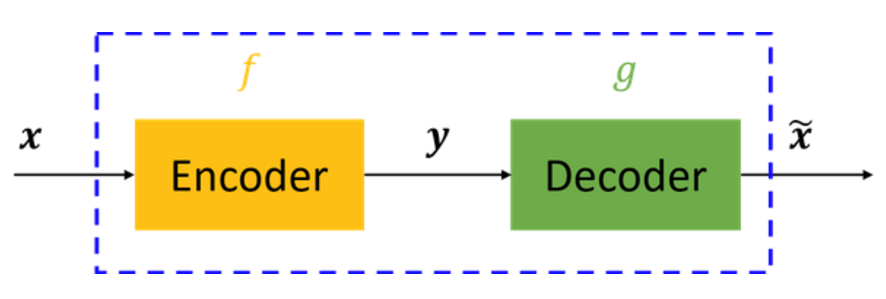图中，虚线蓝色框内就是一个自编码器模型，它由编码器（Encoder）和解码器（Decoder）两部分组成，本质上都是对输入信号做某种变换。编码器将输入信号x变换成编码信号y，而解码器将编码y转换成输出信号\tilde{x}。即
y=f(x)\tilde{x}=g(y)=g(f(x))
而自编码器的目的是，让输出\tilde{x}尽可能复现输入x，即tries to copy its input to its output。但是，这样问题就来了——如果f和g都是恒等映射，那不就恒有\tilde{x}=x了？不错，确实如此，但这样的变换——没有任何卵用啊！因此，我们经常对中间信号y（也叫作“编码”）做一定的约束，这样，系统往往能学出很有趣的编码变换f和编码y。

这里强调一点，对于自编码器，我们往往并不关系输出是啥（反正只是复现输入），我们真正关心的是中间层的编码，或者说是从输入到编码的映射。可以这么想，在我们强迫编码y和输入x不同的情况下，系统还能够去复原原始信号x，那么说明编码y已经承载了原始数据的所有信息，但以一种不同的形式！这就是特征提取啊，而且是自动学出来的！实际上，自动学习原始数据的特征表达也是神经网络和深度学习的核心目的之一。

为了更好的理解自编码器，下面结合神经网络加以介绍。
自编码器与神经网络

神经网络的知识不再详细介绍，相信了解自编码器的读者或多或少会了解一些。简单来讲，神经网络就是在对原始信号逐层地做非线性变换，如下图所示。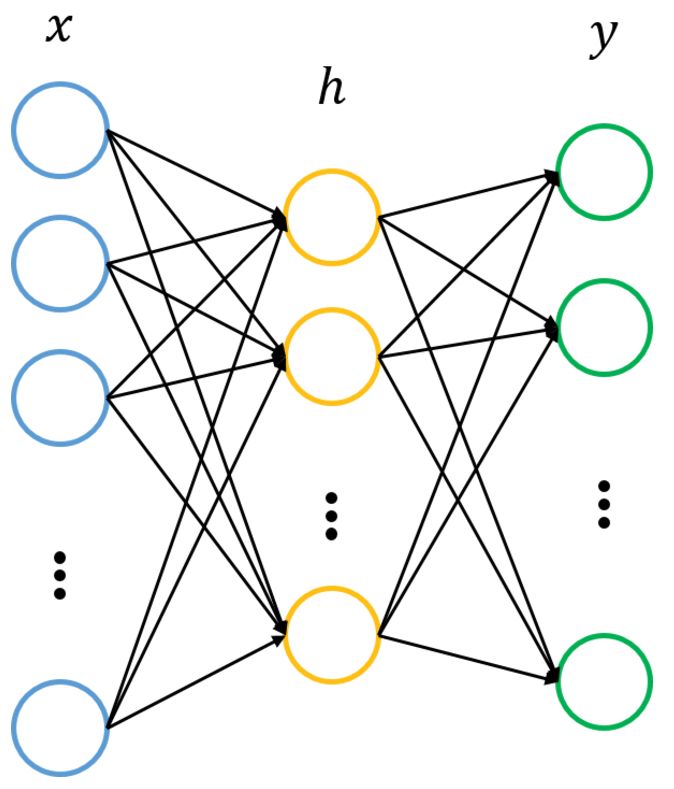该网络把输入层数据x∈Rn转换到中间层（隐层）h∈Rp，再转换到输出层y∈Rm。图中的每个节点代表数据的一个维度（偏置项图中未标出）。每两层之间的变换都是“线性变化”+“非线性激活”，用公式表示即为
h=f(W(1)x+b(1))y=f(W(2)h+b(2))
神经网络往往用于分类，其目的是去逼近从输入层到输出层的变换函数。因此，我们会定义一个目标函数来衡量当前的输出和真实结果的差异，利用该函数去逐步调整（如梯度下降）系统的参数（W(1),b(1),W(2),b(2)），以使得整个网络尽可能去拟合训练数据。如果有正则约束的话，还同时要求模型尽量简单（防止过拟合）。

那么，自编码器怎么表示呢？前面已说过，自编码器试图复现其原始输入，因此，在训练中，网络中的输出应与输入相同，即y=x，因此，一个自编码器的输入、输出应有相同的结构，即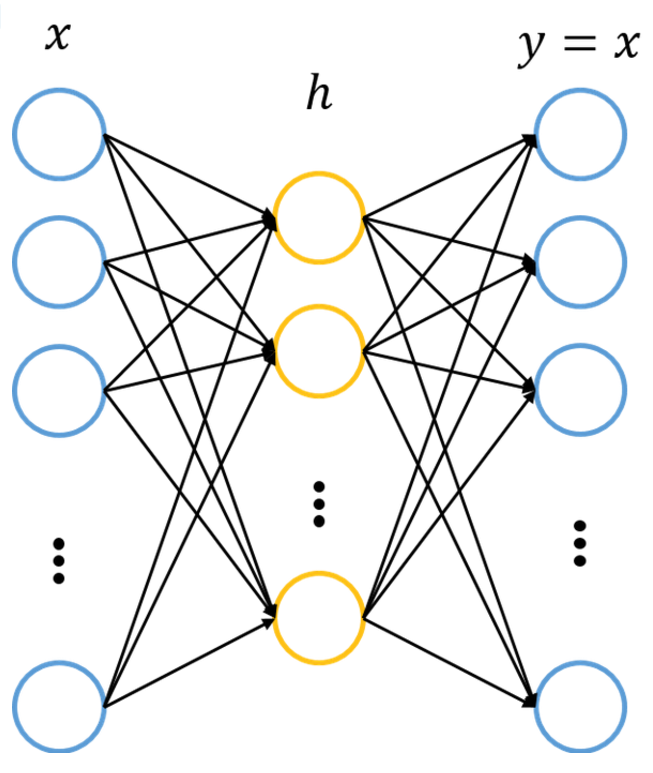我们利用训练数据训练这个网络，等训练结束后，这个网络即学习出了x→h→x的能力。对我们来说，此时的h是至关重要的，因为它是在尽量不损失信息量的情况下，对原始数据的另一种表达。结合神经网络的惯例，我们再将自编码器的公式表示如下：（假设激活函数是sigmoid，用s表示）
y=fθ(x)=s(Wx+b)\tilde{x}=gθ′(y)=s(W′y+b′)L(x,\tilde{x}）=L(x,g(f(x)))
其中，L表示损失函数，结合数据的不同形式，可以是二次误差（squared error loss）或交叉熵误差（cross entropy loss）。如果W^{'}=W^{T}，一般称为tied weights。

为了尽量学到有意义的表达，我们会给隐层加入一定的约束。从数据维度来看，常见以下两种情况：
n>p，即隐层维度小于输入数据维度。也就是说从x→h的变换是一种降维的操作，网络试图以更小的维度去描述原始数据而尽量不损失数据信息。实际上，当每两层之间的变换均为线性，且监督训练的误差是二次型误差时，该网络等价于PCA！没反应过来的童鞋可以反思下PCA是在做什么事情。n<p，即隐层维度大于输入数据维度。这又有什么用呢？其实不好说，但比如我们同时约束h的表达尽量稀疏（有大量维度为0，未被激活），此时的编码器便是大名鼎鼎的“稀疏自编码器”。可为什么稀疏的表达就是好的？这就说来话长了，有人试图从人脑机理对比，即人类神经系统在某一刺激下，大部分神经元是被抑制的。个人觉得，从特征的角度来看更直观些，稀疏的表达意味着系统在尝试去特征选择，找出大量维度中真正重要的若干维。堆叠自编码器

有过深度学习基础的童鞋想必了解，深层网络的威力在于其能够逐层地学习原始数据的多种表达。每一层的都以底一层的表达为基础，但往往更抽象，更加适合复杂的分类等任务。

堆叠自编码器实际上就在做这样的事情，如前所述，单个自编码器通过虚构x→h→x的三层网络，能够学习出一种特征变化h=fθ(x)（这里用θ表示变换的参数，包括W,b和激活函数）。实际上，当训练结束后，输出层已经没什么意义了，我们一般将其去掉，即将自编码器表示为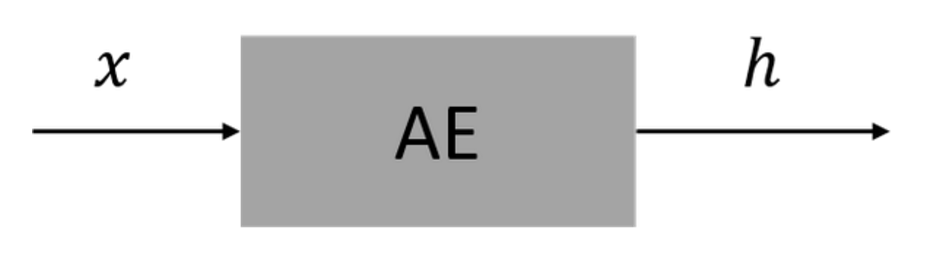之前之所以将自编码器模型表示为3层的神经网络，那是因为训练的需要，我们将原始数据作为假想的目标输出，以此构建监督误差来训练整个网络。等训练结束后，输出层就可以去掉了，我们关心的只是从x到h的变换。

接下来的思路就很自然了——我们已经得到特征表达h，那么我们可不可以将

h再当做原始信息，训练一个新的自编码器，得到新的特征表达呢？当然可以！这就是所谓的堆叠自编码器（Stacked Auto-Encoder, SAE）。Stacked就是逐层垒叠的意思，跟“栈”有点像。UFLDL教程将其翻译为“栈式自编码”，anyway，不管怎么称呼，都是这个东东，别被花里胡哨的专业术语吓到就行。当把多个自编码器Stack起来之后，这个系统看起来就像这样：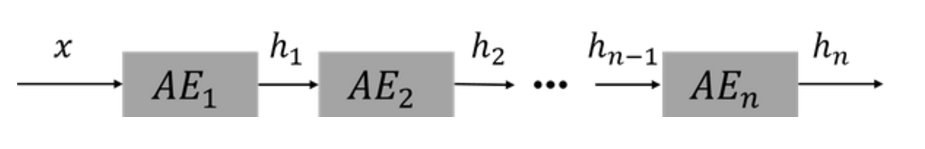亦可赛艇！这个系统实际上已经有点深度学习的味道了，即learning multiple levels of representation and abstraction(Hinton, Bengio, LeCun, 2015)。需要注意的是，整个网络的训练不是一蹴而就的，而是逐层进行。按题主提到的结构n,m,k结构，实际上我们是先训练网络n→m→n，得到n→m的变换，然后再训练m→k→m，得到m→k的变换。最终堆叠成SAE，即为n→m→k的结果，整个过程就像一层层往上盖房子，这便是大名鼎鼎的layer-wise
unsuperwised pre-training（逐层非监督预训练），正是导致深度学习（神经网络）在2006年第3次兴起的核心技术。

关于逐层预训练与深度学习，将在本文最后探讨。
自编码器的变种形式

上述介绍的自编码器是最基本的形式。善于思考的童鞋可能已经意识到了这个问题：隐层的维度到底怎么确定？为什么稀疏的特征比较好？或者更准确的说，怎么才能称得上是一个好的表达（What defines a good representation）？

事实上，这个问题回答并不唯一，也正是从不同的角度去思考这个问题，导致了自编码器的各种变种形式出现。目前常见的几种模型总结如下（有些术语实在不好翻译，看英文就好。。。）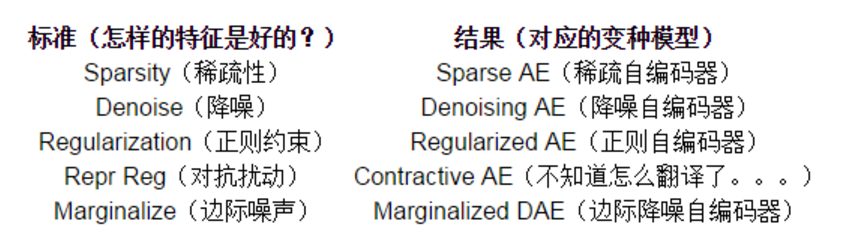下面简介下其中两种模型，以对这些变种模型有个直观感受。
稀疏自编码器

UFLDL-自编码算法与稀疏性对该模型有着比较详细的介绍。如前所示，这种模型背后的思想是，高维而稀疏的表达是好的。一般而言，我们不会指定隐层表达h中哪些节点是被抑制的（对于sigmoid单元即输出为0），而是指定一个稀疏性参数ρ，代表隐藏神经元的平均活跃程度（在训练集上取平均）。比如，当ρ=0.05时，可以认为隐层节点在95%的时间里都是被抑制的，只有5%的机会被激活。实际上，为了满足这一条件，隐层神经元的活跃度需要接近于0。

那么，怎么从数学模型上做到这点呢？思路也不复杂，既然要求平均激活度为ρ，那么只要引入一个度量，来衡量神经元ii的实际激活度\tilde{ρ_{a}}与期望激活度ρ之间的差异即可，然后将这个度量添加到目标函数作为正则，训练整个网络即可。那么，什么样的度量适合这个任务呢？有过概率论、信息论基础的同学应该很容易想到它——相对熵，也就是KL散度（KL
divergence）。因此，整个网络所添加的惩罚项即为
\sum_{i\in h}{KL(ρ || \tilde{ρ_{a}})}
具体的公式不再展开，可以从下图（摘自UFLDL）中直观理解KL散度作为惩罚项的含义。图中假设平均激活度ρ=0.2。可以看出，当^ρiρ^i一旦偏离期望激活度ρρ，这种误差便急剧增大，从而作为惩罚项添加到目标函数，指导整个网络学习出稀疏的特征表达。
降噪自编码器

关于降噪自编码器，强烈推荐其作者Pascal Vincent的论文Stacked Denoising Autoencoders: Learning Useful Representations in a Deep Network with a Local Denoising Criterion。DAE的核心思想是，一个能够从中恢复出原始信号的表达未必是最好的，能够对“被污染/破坏”的原始数据编码、解码，然后还能恢复真正的原始数据，这样的特征才是好的。

稍微数学一点，假设原始数据x被我们“故意破坏”，比如加入高斯白噪，或者把某些维度数据抹掉，变成了\bar{X}，然后再对\bar{X}编码、解码，得到恢复信号\tilde{X}=g(f(X))，该恢复信号尽可能逼近未被污染的数据xx。此时，监督训练的误差从L(x,g(f(x)))变成了L(x,g(f(\TILDE{X})))。

直观上理解，DAE希望学到的特征变换尽可能鲁棒，能够在一定程度上对抗原始数据的污染、缺失。Vincent论文里也对DAE提出了基于流行的解释，并且在图像数据上进行测试，发现DAE能够学出类似Gabor边缘提取的特征变换。注意，这一切都是在我们定义好规则、误差后，系统自动学出来的！从而避免了领域专家费尽心力去设计这些性能良好的特征。

DAE的系统结构如下图（摘自Vincent论文）所示。%%%%%%%%%%%%%%%%%%%%%%%%

其他的模型就不再展开了，总之，每遇到一个自编码器的一个变种模型时，搞清楚其背后的思想（什么样的表达才是好的），就很容易掌握了。套用V的”Behind this mask is a man, and behind this man is an idea, and ideas are bulletproof”，我们可以说，”Behind this auto-encoder is a model, and behind this model is an idea, and ideas are bulletproof”。
关于预训练与深度学习

深度学习第3次兴起正式因为逐层预训练方法的提出，使得深度网络的训练成为可能。对于一个深度网络，这种逐层预训练的方法，正是前面介绍的这种Stacked Auto-Encoder。对于常见的分类任务，一般分为以下两个阶段：
layer-wise pre-training （逐层预训练）fune-tuning （微调）
注意到，前述的各种SAE，本质上都是非监督学习，SAE各层的输出都是原始数据的不同表达。对于分类任务，往往在SAE顶端再添加一分类层（如Softmax层），并结合有标注的训练数据，在误差函数的指导下，对系统的参数进行微调，以使得整个网络能够完成所需的分类任务。对于微调过程，即可以只调整分类层的参数（此时相当于把整个SAE当做一个feature extractor），也可以调整整个网络的参数（适合训练数据量比较大的情况）。

为什么训练稀疏自编码器为什么一般都是3层的结构？实际上这里的3层是指训练单个自编码器所假想的3层神经网络，这对任何基于神经网络的编码器都是如此。多层的稀疏自编码器自然是有的，只不过是通过layer-wise pre-training这种方式逐层垒叠起来的，而不是直接去训练一个5层或是更多层的网络。

为什么要这样？实际上，这正是在训练深层神经网络中遇到的问题。直接去训练一个深层的自编码器，其实本质上就是在做深度网络的训练，由于梯度扩散等问题，这样的网络往往根本无法训练。这倒不是因为会破坏稀疏性等原因，只要网络能够训练，对模型施加的约束总能得到相应的结果。

但为什么逐层预训练就可以使得深度网络的训练成为可能了呢？有不少文章也做过这方面的研究。一个直观的解释是，预训练好的网络在一定程度上拟合了训练数据的结构，这使得整个网络的初始值是在一个合适的状态，便于有监督阶段加快迭代收敛。

笔者曾经基于 MNIST数据集，尝试了一个9层的网络完成分类任务。当随机初始化时，误差传到底层几乎全为0，根本无法训练。但采用逐层预训练的方法，训练好每两层之间的自编码变换，将其参数作为系统初始值，然后网络在有监督阶段就能比较稳定的迭代了。

当然，有不少研究提出了很好的初始化策略，再加上现在常用的dropout、ReLU，直接去训练一个深层网络已经不是问题。这是否意味着这种逐层预训练的方式已经过时了呢？这里，我想采用下Bengio先生2015年的一段话作为回答：

Stacks of unsupervised feature learning layers are STILL useful when you are in a regime with insufficient labeled examples, for transfer learning or domain adaptation. It is a regularizer. But when the number of labeled examples becomes large enough, the advantage
of that regularizer becomes much less. I suspect however that this story is far from ended! There are other ways besides pre-training of combining supervised and unsupervised learning, and I believe that we still have a lot to improve in terms of our unsupervised
learning algorithms.

最后，多说一句，除了AE和SAE这种逐层预训练的方式外，还有另外一条类似的主线，即限制玻尔兹曼机（RBM）与深度信念网络（DBN）。这些模型在神经网络/深度学习框架中的位置，可以简要总结为下图。[MLP] -> http://blog.csdn.net/cxf7394373/article/details/6061372    [FFNN] -> feedforward neural network 前馈神经网络

相关学习资料推荐
Sranford UFLDL教程 旧版有中文版作为参考Deep Learning Tutorial (Theano) 其中有关于AE、DAE、SDAE基于Theano的实现DeepLearnToolbox 该Toolbox基于Matlab实现，其中有SAE、CAE的实现相关论文Hinton, G.E. and R.R. Salakhutdinov, Reducing the dimensionality of data with neural networks. Science, 2006. 313(5786): p. 504-507.Learning multiple layers of representation. Trends in cognitive sciences, 2007. 11(10): p. 428-434.Vincent, P., et al. Extracting and composing robust features with denoising autoencoders. in Proceedings of the 25th international conference on Machine learning. 2008.Bengio, Y., Learning deep architectures for AI. Foundations and trends? in Machine Learning, 2009. 2(1): p. 1-127.Vincent, P., et al., Stacked Denoising Autoencoders: Learning Useful Representations in a Deep Network with a Local Denoising Criterion. Journal of Machine Learning Research, 2010. 11(6): p. 3371-3408.Rifai, S., et al., Contractive Auto-Encoders: Explicit Invariance During Feature Extraction. Icml, 2011.Chen, M., et al., Marginalized denoising autoencoders for domain adaptation. arXiv preprint arXiv:1206.4683, 2012.Bengio, Y., A. Courville and P. Vincent, Representation learning: A review and new perspectives. Pattern Analysis and Machine Intelligence, IEEE Transactions on, 2013. 35(8): p. 1798-1828.LeCun, Y., Y. Bengio and G. Hinton, Deep learning. Nature, 2015. 521(7553): p. 436-444.

展开全文• 自编码器（Autoencoder,AE）,是一种利用反向传播算法使得输出值等于输入值的神经网络，它先将输入压缩成潜在空间表征，然后通过这种表征来重构输出。自编码器由两部分组成：编码器（encoder）:这部分能将输入压缩成...
自编码器（Autoencoder,AE）,是一种利用反向传播算法使得输出值等于输入值的神经网络，它先将输入压缩成潜在空间表征，然后通过这种表征来重构输出。

自编码器由两部分组成：

编码器（encoder）:这部分能将输入压缩成潜在空间表征，可以用编码函数h=f(x)表示。

解码器（decoder）:这部分重构来自潜在空间表征的输入，可以用解码函数r=g(h)表示。

因此，整个自编码器可以用函数g(f(x)) = r 来描述，其中输出r与原始输入x相近。

一、为何要用输入来重构输出？

如果自编码器的唯一目的是让输出值等于输入值，那种人个算法将毫无用处。事实上，我们希望通过训练输出值等于输入值的自编码器，让潜在表征h将具有价值属性。

这可通过在重构任务中构建约束来实现。

从自编码器获得有用特征的一种方法是，限制h的维度使其小于输入x,这种情况下称作有损自编码器。通过训练有损表征，使得自编码器能学习到数据中最重要的特征。

如果潜在表征的维度与输入相同，或是在完备案例中潜在表征的维度大于输入，上述结果也会出现。

在这些情况下，即使只使用线性编码器和线性解码器，也能很好地利用输入重构输出，且无需了解有关数据分布的任何有用信息。

在理想情况下，根据要分配的数据复杂度，来准确选择编码器和解码器的编码维数和容量，就可以成功地训练出任何所需的自编码器结构。

二、自编码器用来干什么？

目前，自编码器的应用主要有两个方面：

1.第一是数据去噪

2.第二是为进行可视化而降维。

设置合适的维度和稀疏约束，自编码器可以学习到PCA等技术更有意思的数据投影。

自编码器能从数据样本中进行无监督学习，这意味着可以将这个算法应用到某个数据集中，来取得良好的性能，且不需要任何新的特征工程，只需要适当地训练数据。

但是，自编码器在图像压缩方面表现的不好。由于在某个给定数据集上训练自编码器，因此它在处理与训练集相类似的数据时可达到合理的压缩结果，但是在压缩差异较大的其他图像时效果不佳。这里，像JPEG这样的压缩技术在通用图像压缩方面会表现得更好。

训练自编码器，可以使输入通过编码器和解码器后，保留尽可能多的信息，但也可以训练自编码器来使新表征具有多种不同的属性。不同类型的自编码器旨在实现不同类型的属性。下面将重点介绍四中不同的自编码器。

三、四种不同的自编码器

本文将介绍以下四种不同的自编码器：

vanilla自编码器

多层自编码器

卷积自编码器

正则自编码器

vanilla自编码器

在这里，如果隐含层维数（64）小于输入维数（784），则称这个编码器是有损的。通过这个约束，来迫使神经网络来学习数据的压缩表征。

input_size = 784
hidden_size = 64
output_size = 784

x = Input(shape=(input_size,))

# Encoder
h = Dense(hidden_size, activation='relu')(x)

# Decoder
r = Dense(output_size, activation='sigmoid')(h)

autoencoder = Model(input=x, output=r)
autoencoder.compile(optimizer='adam', loss='mse')

多层自编码器

如果一个隐含层还不够，显然可以将自动编码器的隐含层数目进一步提高。

在这里，实现中使用了3个隐含层，而不是只有一个。任意一个隐含层都可以作为特征表征，但是为了使网络对称，我们使用了最中间的网络层。

input_size = 784
hidden_size = 128
code_size = 64

x = Input(shape=(input_size,))

# Encoder
hidden_1 = Dense(hidden_size, activation='relu')(x)
h = Dense(code_size, activation='relu')(hidden_1)

# Decoder
hidden_2 = Dense(hidden_size, activation='relu')(h)
r = Dense(input_size, activation='sigmoid')(hidden_2)

autoencoder = Model(input=x, output=r)
autoencoder.compile(optimizer='adam', loss='mse')

卷积自编码器

你可能有个疑问，除了全连接层，自编码器应用到卷积层吗？

答案是肯定的，原理是一样的，但是要使用3D矢量（如图像）而不是展平后的一维矢量。对输入图像进行下采样，以提供较小维度的潜在表征，来迫使自编码器从压缩后的数据进行学习。

x = Input(shape=(28, 28,1))

# Encoder
conv1_1 = Conv2D(16, (3, 3), activation='relu', padding='same')(x)
conv1_2 = Conv2D(8, (3, 3), activation='relu', padding='same')(pool1)
conv1_3 = Conv2D(8, (3, 3), activation='relu', padding='same')(pool2)

# Decoder
conv2_1 = Conv2D(8, (3, 3), activation='relu', padding='same')(h)
up1 = UpSampling2D((2, 2))(conv2_1)
conv2_2 = Conv2D(8, (3, 3), activation='relu', padding='same')(up1)
up2 = UpSampling2D((2, 2))(conv2_2)
conv2_3 = Conv2D(16, (3, 3), activation='relu')(up2)
up3 = UpSampling2D((2, 2))(conv2_3)
r = Conv2D(1, (3, 3), activation='sigmoid', padding='same')(up3)

autoencoder = Model(input=x, output=r)
autoencoder.compile(optimizer='adam', loss='mse')

正则自编码器

除了施加一个比输入维度小的隐含层，一些其他方法也可用来约束自编码器重构，如正则自编码器。

正则自编码器不需要使用浅层的编码器和解码器以及小的编码维数来限制模型容量，而是使用损失函数来鼓励模型学习其他特性（除了将输入复制到输出）。这些特性包括稀疏表征、小导数表征、以及对噪声或输入缺失的鲁棒性。

即使模型容量大到足以学习一个无意义的恒等函数，非线性且过完备的正则自编码器仍然能够从数据中学到一些关于数据分布的有用信息。

在实际应用中，常用到两种正则自编码器，分别是稀疏自编码器和降噪自编码器。

稀疏自编码器：

一般用来学习特征，以便用于像分类这样的任务。稀疏正则化的自编码器必须反映训练数据集的独特统计特征，而不是简单地充当恒等函数。以这种方式训练，执行附带稀疏惩罚的复现任务可以得到能学习有用特征的模型。

还有一种用来约束自动编码器重构的方法，是对其损失函数施加约束。比如，可对损失函数添加一个正则化约束，这样能使自编码器学习到数据的稀疏表征。

要注意，在隐含层中，我们还加入了L1正则化，作为优化阶段中损失函数的惩罚项。与香草自编码器相比，这样操作后的数据表征更为稀疏。

input_size = 784
hidden_size = 64
output_size = 784

x = Input(shape=(input_size,))

# Encoder
h = Dense(hidden_size, activation='relu', activity_regularizer=regularizers.l1(10e-5))(x)#施加在输出上的L1正则项

# Decoder
r = Dense(output_size, activation='sigmoid')(h)

autoencoder = Model(input=x, output=r)
autoencoder.compile(optimizer='adam', loss='mse')

降噪自编码器：

这里不是通过对损失函数施加惩罚项，而是通过改变损失函数的重构误差项来学习一些有用信息。

向训练数据加入噪声，并使自编码器学会去除这种噪声来获得没有被噪声污染过的真实输入。因此，这就迫使编码器学习提取最重要的特征并学习输入数据中更加鲁棒的表征，这也是它的泛化能力比一般编码器强的原因。

这种结构可以通过梯度下降算法来训练。

x = Input(shape=(28, 28, 1))

# Encoder
conv1_1 = Conv2D(32, (3, 3), activation='relu', padding='same')(x)
conv1_2 = Conv2D(32, (3, 3), activation='relu', padding='same')(pool1)

# Decoder
conv2_1 = Conv2D(32, (3, 3), activation='relu', padding='same')(h)
up1 = UpSampling2D((2, 2))(conv2_1)
conv2_2 = Conv2D(32, (3, 3), activation='relu', padding='same')(up1)
up2 = UpSampling2D((2, 2))(conv2_2)
r = Conv2D(1, (3, 3), activation='sigmoid', padding='same')(up2)

autoencoder = Model(input=x, output=r)
autoencoder.compile(optimizer='adam', loss='mse')

使用tensorflow实现对手写字(MNIST)的AutoEncoder

import tensorflow as tf
import numpy as np
import matplotlib.pyplot as plt

# Import MNIST data
from tensorflow.examples.tutorials.mnist import input_data

# Visualize decoder setting
# Parameters
learning_rate = 0.01
batch_size = 256
display_step = 1
examples_to_show = 10

# Network Parameters
n_input = 784  # 28x28 pix，即 784 Features

# tf Graph input (only pictures)
X = tf.placeholder("float", [None, n_input])

# hidden layer settings
n_hidden_1 = 256 # 经过第一个隐藏层压缩至256个
n_hidden_2 = 128 # 经过第二个压缩至128个
#两个隐藏层的 weights 和 biases 的定义
weights = {
'encoder_h1': tf.Variable(tf.random_normal([n_input, n_hidden_1])),
'encoder_h2': tf.Variable(tf.random_normal([n_hidden_1, n_hidden_2])),
'decoder_h1': tf.Variable(tf.random_normal([n_hidden_2, n_hidden_1])),
'decoder_h2': tf.Variable(tf.random_normal([n_hidden_1, n_input])),
}
biases = {
'encoder_b1': tf.Variable(tf.random_normal([n_hidden_1])),
'encoder_b2': tf.Variable(tf.random_normal([n_hidden_2])),
'decoder_b1': tf.Variable(tf.random_normal([n_hidden_1])),
'decoder_b2': tf.Variable(tf.random_normal([n_input])),
}

# Building the encoder
def encoder(x):
# Encoder Hidden layer 使用的 Activation function 是 sigmoid #1
biases['encoder_b1']))
# Decoder Hidden layer with sigmoid activation #2
biases['encoder_b2']))
return layer_2

# Building the decoder
def decoder(x):
# Encoder Hidden layer with sigmoid activation #1
biases['decoder_b1']))
# Decoder Hidden layer with sigmoid activation #2
biases['decoder_b2']))
return layer_2

'''

# Visualize encoder setting
# 只显示解压后的数据
learning_rate = 0.01    # 0.01 this learning rate will be better! Tested
training_epochs = 10
batch_size = 256
display_step = 1
# Network Parameters
n_input = 784  # MNIST data input (img shape: 28*28)
# tf Graph input (only pictures)
X = tf.placeholder("float", [None, n_input])
# hidden layer settings
n_hidden_1 = 128
n_hidden_2 = 64
n_hidden_3 = 10
n_hidden_4 = 2  #将原有784Features 的数据压缩成2 Features数据
weights = {
'encoder_h1': tf.Variable(tf.truncated_normal([n_input, n_hidden_1],)),
'encoder_h2': tf.Variable(tf.truncated_normal([n_hidden_1, n_hidden_2],)),
'encoder_h3': tf.Variable(tf.truncated_normal([n_hidden_2, n_hidden_3],)),
'encoder_h4': tf.Variable(tf.truncated_normal([n_hidden_3, n_hidden_4],)),
'decoder_h1': tf.Variable(tf.truncated_normal([n_hidden_4, n_hidden_3],)),
'decoder_h2': tf.Variable(tf.truncated_normal([n_hidden_3, n_hidden_2],)),
'decoder_h3': tf.Variable(tf.truncated_normal([n_hidden_2, n_hidden_1],)),
'decoder_h4': tf.Variable(tf.truncated_normal([n_hidden_1, n_input],)),
}
biases = {
'encoder_b1': tf.Variable(tf.random_normal([n_hidden_1])),
'encoder_b2': tf.Variable(tf.random_normal([n_hidden_2])),
'encoder_b3': tf.Variable(tf.random_normal([n_hidden_3])),
'encoder_b4': tf.Variable(tf.random_normal([n_hidden_4])),
'decoder_b1': tf.Variable(tf.random_normal([n_hidden_3])),
'decoder_b2': tf.Variable(tf.random_normal([n_hidden_2])),
'decoder_b3': tf.Variable(tf.random_normal([n_hidden_1])),
'decoder_b4': tf.Variable(tf.random_normal([n_input])),#注意：在第四层时，输出量不再是 [0,1] 范围内的数，
#而是将数据通过默认的 Linear activation function 调整为 (-∞,∞)
}
def encoder(x):
biases['encoder_b1']))
biases['encoder_b2']))
biases['encoder_b3']))
biases['encoder_b4'])
return layer_4
def decoder(x):
biases['decoder_b1']))
biases['decoder_b2']))
biases['decoder_b3']))
biases['decoder_b4']))
return layer_4
'''

# Construct model
encoder_op = encoder(X)
decoder_op = decoder(encoder_op)

# Prediction
y_pred = decoder_op
# Targets (Labels) are the input data.
y_true = X

# Define loss and optimizer, minimize the squared error
#比较原始数据与还原后的拥有 784 Features 的数据进行 cost 的对比，
#根据 cost 来提升我的 Autoencoder 的准确率
loss = tf.reduce_mean(tf.pow(y_true - y_pred, 2))#进行最小二乘法的计算(y_true - y_pred)^2
#loss = tf.reduce_mean(tf.square(y_true - y_pred))

# Launch the graph
with tf.Session() as sess:
init = tf.global_variables_initializer()
sess.run(init)
total_batch = int(mnist.train.num_examples/batch_size)
training_epochs = 20
# Training cycle
for epoch in range(training_epochs):#到好的的效果，我们应进行10 ~ 20个 Epoch 的训练
# Loop over all batches
for i in range(total_batch):
batch_xs, batch_ys = mnist.train.next_batch(batch_size)  # max(x) = 1, min(x) = 0
# Run optimization op (backprop) and cost op (to get loss value)
_, c = sess.run([optimizer, loss], feed_dict={X: batch_xs})
# Display logs per epoch step
if epoch % display_step == 0:
print("Epoch:", '%04d' % (epoch+1),
"cost=", "{:.9f}".format(c))
print("Optimization Finished!")

# Applying encode and decode over test set
encode_decode = sess.run(
y_pred, feed_dict={X: mnist.test.images[:examples_to_show]})
# Compare original images with their reconstructions
f, a = plt.subplots(2, 10, figsize=(10, 2))
for i in range(examples_to_show):
a[i].imshow(np.reshape(mnist.test.images[i], (28, 28)))
a[i].imshow(np.reshape(encode_decode[i], (28, 28)))

plt.show()

# encoder_result = sess.run(encoder_op, feed_dict={X: mnist.test.images})
# sc = plt.scatter(encoder_result[:, 0], encoder_result[:, 1], c=mnist.test.labels) #散点图
# plt.colorbar(sc) #scatter设置颜色渐变条colorbar
# plt.show()

上面一行图片为测试图片，第二行为经过自编码器后输出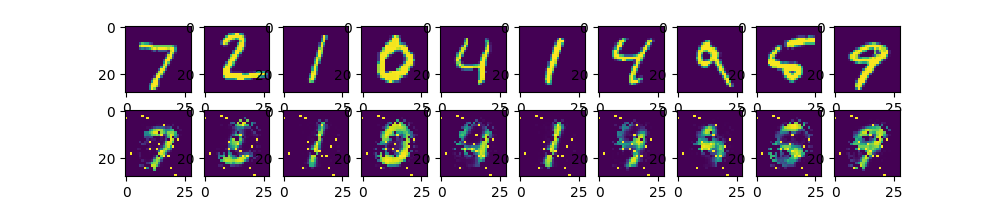图1 手写数字照片经自编码器输出与原照片对比

应用--基于降噪自编码器的情感分析

一个简单的降噪自动编码器如图2( a) 所示，最底层的小圆代表原始数据而带淡蓝色的小圆则是噪声数据。将这些数据输入到编码器中会得到输入数据的一个表示，再将这个表示通过解码器输出一个信息，通过调整编码器和解码器的参数使得重构误差最小。

将上一层的输出作为下一层的输入，其逐层嵌入可如图2( b) 所示。为了实现情感分析的任务，必须在输出层的前一层添加一个分类器softmax 层，然后通过标准的多层神经网络监督训练梯度下降法，最后得到如图2( c) 中的堆叠多隐层的降噪自动编码器。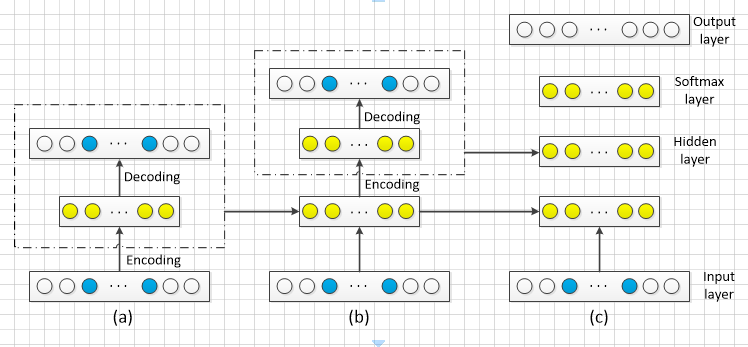图2 降噪自动编码器的逐层嵌入与训练模型

总结

本文先介绍了自编码器的基本结构，还研究了许多不同类型的自编码器，如vanilla、多层、卷积和正则化，通过施加不同的约束，包括缩小隐含层的维度加入惩罚项，使得每种自编码器具有不同属性。

希望这篇文章能让深度学习初学者对自编码器有个很好的认识。


展开全文深度学习
• 自编码器是一种能够通过无监督学习，学到输入数据高效表示的人工神经网络。输入数据的这一高效表示称为编码（codings），其维度一般远小于输入数据，使得自编码器可用于降维。更重要的是，自编码器可作为强大的特征...
   自编码器是一种能够通过无监督学习，学到输入数据高效表示的人工神经网络。输入数据的这一高效表示称为编码（codings），其维度一般远小于输入数据，使得自编码器可用于降维。更重要的是，自编码器可作为强大的特征检测器（feature detectors），应用于深度神经网络的预训练。此外，自编码器还可以随机生成与训练数据类似的数据，这被称作生成模型（generative model）。比如，可以用人脸图片训练一个自编码器，它可以生成新的图片。

自编码器通过简单地学习将输入复制到输出来工作。这一任务（就是输入训练数据， 再输出训练数据的任务）听起来似乎微不足道，但通过不同方式对神经网络增加约束，可以使这一任务变得极其困难。比如，可以限制内部表示的尺寸（这就实现降维了），或者对训练数据增加噪声并训练自编码器使其能恢复原有。这些限制条件防止自编码器机械地将输入复制到输出，并强制它学习数据的高效表示。简而言之，编码（就是输入数据的高效表示）是自编码器在一些限制条件下学习恒等函数（identity function）的副产品。

1.高效的数据表示

下面有两组数字，哪组更容易记忆呢？

40, 27, 25, 36, 81, 57, 10, 73, 19, 68
50, 25, 76, 38, 19, 58, 29, 88, 44, 22, 11, 34, 17, 52, 26, 13, 40, 20
乍一看可能觉得第一行数字更容易记忆，毕竟更短。但仔细观察就会发现，第二组数字是有规律的：偶数后面是其二分之一，奇数后面是其三倍加一（这就是著名的hailstone sequence）。如果识别出了这一模式，第二组数据只需要记住这两个规则、第一个数字、以及序列长度。如果你的记忆能力超强，可以记住很长的随机数字序列，那你可能就不会去关心一组数字是否存在规律了。所以我们要对自编码器增加约束来强制它去探索数据中的模式。

记忆（memory）、感知（perception）、和模式匹配（pattern matching）的关系在1970s早期就被William Chase和Herbert Simon研究过。他们发现国际象棋大师观察棋盘5秒，就能记住所有棋子的位置，而常人是无法办到的。但棋子的摆放必须是实战中的棋局（也就是棋子存在规则，就像第二组数字），棋子随机摆放可不行（就像第一组数字）。象棋大师并不是记忆力优于我们，而是经验丰富，很擅于识别象棋模式，从而高效地记忆棋局。

和棋手的记忆模式类似，一个自编码器接收输入，将其转换成高效的内部表示，然后再输出输入数据的类似物。自编码器通常包括两部分：encoder（也称为识别网络）将输入转换成内部表示，decoder（也称为生成网络）将内部表示转换成输出。（如图1）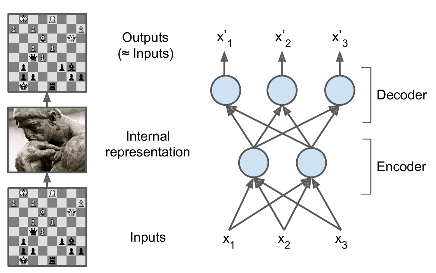\

图1 象棋大师的记忆模式（左）和一个简单的自编码器

正如上图所示，自编码器的结构和多层感知机类似，除了输入神经元和输出神经元的个数相等。在上图的例子中，自编码器只有一个包含两个神经元的隐层（encoder），以及包含3个神经元的输出层（decoder）。输出是在设法重建输入，损失函数是重建损失（reconstruction loss）。

由于内部表示（也就是隐层的输出）的维度小于输入数据（用2D取代了原来的3D),这称为不完备自编码器（undercomplete autoencoder）。

2 .不完备线性自编码器实现PCA（Performing PCA with an Undercomplete Linear Autoencoder）

如果自编码器使用线性激活函数并且损失函数是均方差（Mean Squared Error，MSE），那它就可以用来实现主成分分析

下面的代码实现了一个简单的线性自编码器，将3D数据投影为2D：

1

2

3

4

5

6

7

8

9

10

11

12

13

14

15

16

17

18

19

import tensorflow as tf

from tensorflow.contrib.layers import fully_connected

n_inputs = 3 # 3D inputs

n_hidden = 2 # 2D codings

n_outputs = n_inputs

learning_rate = 0.01

X = tf.placeholder(tf.float32, shape=[None, n_inputs])

hidden = fully_connected(X, n_hidden, activation_fn=None)

outputs = fully_connected(hidden, n_outputs, activation_fn=None)

reconstruction_loss = tf.reduce_mean(tf.square(outputs - X)) # MSE

optimizer = tf.train.AdamOptimizer(learning_rate)

training_op = optimizer.minimize(reconstruction_loss)

init = tf.global_variables_initializer()

然后载入数据集，在训练集上训练模型，并对测试集进行编码（也就是投影为2D）：

1

2

3

4

5

6

7

8

9

10

X_train, X_test = [...] # load the dataset

n_iterations = 1000

codings = hidden # the output of the hidden layer provides the codings

with tf.Session() as sess:

    init.run()

    for iteration in range(n_iterations):

        training_op.run(feed_dict={X: X_train}) # no labels (unsupervised)

    codings_val = codings.eval(feed_dict={X: X_test})

3. 栈式自编码器（Stacked Autoencoders）

和其他的神经网络一样，自编码器可以有多个隐层，这被称作栈式自编码器（或者深度自编码器）。增加隐层可以学到更复杂的编码，但千万不能使自编码器过于强大。想象一下，一个encoder过于强大，它仅仅是学习将输入映射为任意数（然后decoder学习其逆映射）。很明显这一自编码器可以很好的重建数据，但它并没有在这一过程中学到有用的数据表示。（而且也不能推广到新的实例）

栈式自编码器的架构一般是关于中间隐层对称的，如图2所示。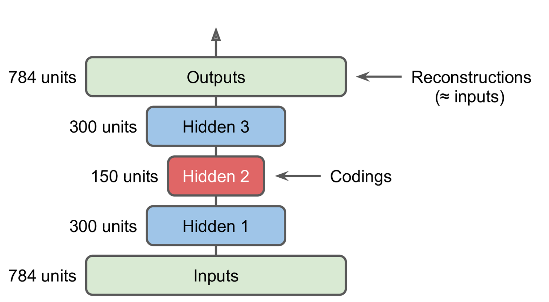图2  栈式自编码器

捆绑权重

如果一个自编码器的层次是严格轴对称的（如图2），一个常用的技术是将decoder层的权重捆绑到encoder层。这使得模型参数减半，加快了训练速度并降低了过拟合风险。

注意：偏置项不会捆绑。

一次训练一个自编码器

与之前训练整个栈式自编码器不同，可以训练多个浅层的自编码器，然后再将它们合并为一体，这样要快得多。如图3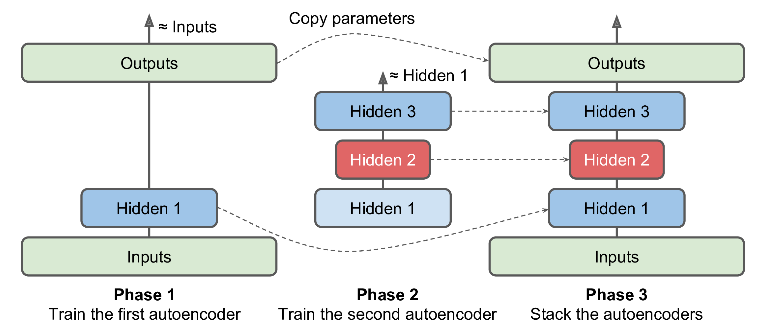图3  一次训练一个浅层自编码器

首先，第一个自编码器学习去重建输入。然后，第二个自编码器学习去重建第一个自编码器隐层的输出。最后，这两个自编码器被整合到一起，如图3。可以使用这种方式，创建一个很深的栈式自编码器。

另一个实现方法首先创建一个包含完整栈式编码器的图，然后再每一个训练时期增加额外的操作，如图4：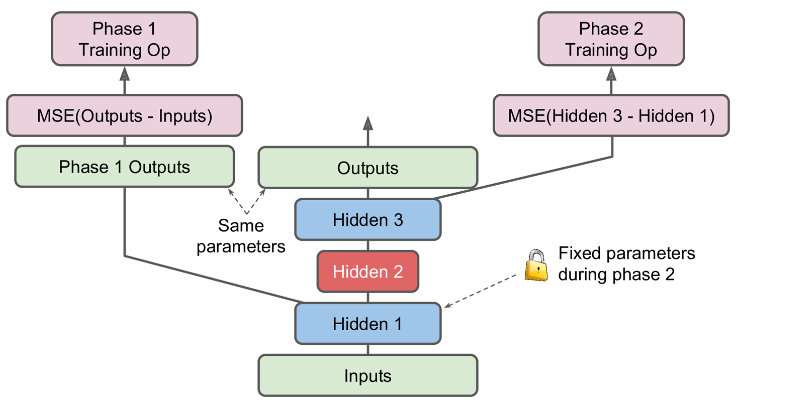图4

其中，

中间的一列是完整的栈式编码器，这部分在训练完成之后可以使用。
左侧一列是最先需要训练的，它跳过第二和第三个隐层，直接创建一个输出层。这个输出层与栈式自编码器的输出层共享同样的权重和偏置。
随后是右侧一列的训练。它使得第三个隐层的输出与第一个隐层的输出尽可能的接近。
4 使用栈式自编码器进行无监督预训练

如果我们要处理一个复杂的有监督学习问题又没有足够的标注数据，一个解决方案是找到一个解决类似任务的训练好的模型，复用低层。类似的，如果有一个很大的数据集但绝大部分是未标注数据，可以使用所有的数据先训练一个栈式自编码器，然后复用低层来完成真正的任务。如图5所示：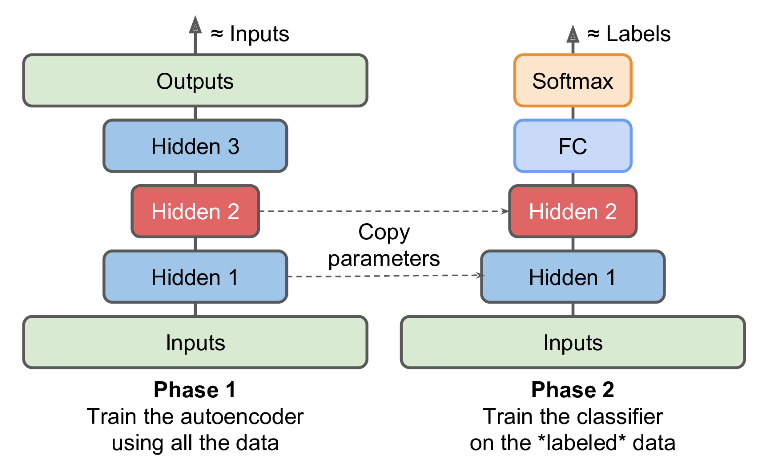图5 使用自编码器进行无监督预训练

5 .去噪自编码器

另一种强制自编码器学习有用特征的方式是最输入增加噪声，通过训练之后得到无噪声的输出。这防止了自编码器简单的将输入复制到输出，从而提取出数据中有用的模式。如图6左侧所示。

噪声可以是添加到输入的纯高斯噪声，也可以是随机丢弃输入层的某个特征，类似于dropout。如图6右侧所示。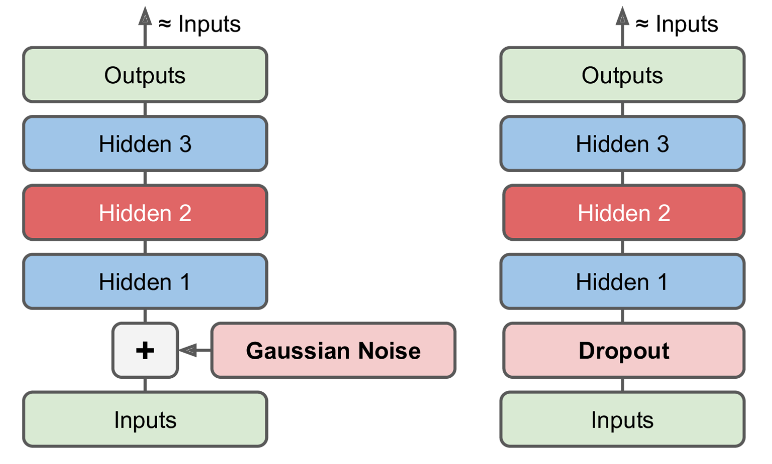图 6  图中自编码器，通过高斯噪声（左）或者Dropout（右）

转自：https://www.cnblogs.com/royhoo/p/Autoencoders.html


展开全文• 一、什么是自编码器（Autoencoder）encoder：编码器；译码器 decoder：解码器；译码器 original input：原输入；初始数据流compressed representation：压缩表示reconstructed input：重新输入自动编码器是一种数据...
• 3.自编码器是什么？有什么用？这里有一份入门指南（附代码） 4.自动编码器 5.自编码器实现代码（可视化版本） 一、稀疏编码 1.生物学背景 稀疏编码（Sparse Coding）也是一种受哺乳动物视觉系统中简单细胞感受野而...
•   在非监督学习中，最典型的一类神经网络莫过于autoencoder(自编码器)，它的目的是基于输入的unlabeled数据X={x(1),x(2),x(3),...}X={x(1),x(2),x(3),...}X=\{x^{(1)}, x^{(2)}, x^{(3)}, ...\}，通过训练得到数据...tensorflow
• 自编码器（Autoencoder，AE）   基本意思就是一个隐藏层的神经网络，输入输出都是x，并且输入维度一定要比输出维度大，属于无监督学习。一种利用反向传播算法使得输出值等于输入值的神经网络，它先将输入压缩成...
• ## 自编码器

千次阅读 2019-07-01 15:36:08
自编码器 在网上一直在搜自编码器的相关资料，但好多看不懂，可能是自己水平限制吧，毕竟对自编码器什么都不懂。经过自己这几天搜集资料，希望能够写一篇相对完善的关于自编码器的资料，希望能有小白看过之后对...
• 自编码器及其变形很多，本篇博客目前主要基于普通自编码器、欠完备自编码器、稀疏自编码器和去噪自编码器，会提供理论+实践（有的理论本人没有完全理解，就先没有写上，后更）。另外，关于收缩自编码器、变分自编码...
• ## 自动编码器

万次阅读 2017-06-07 16:36:24
自动编码器 Deep Learning最简单的一种方法是利用人工神经网络的特点，人工神经网络（ANN）本身就是具有层次结构的系统，如果给定一个神经网络，我们假设其输出与输入是相同的，然后训练调整其参数，得到每一层...
• 自编码器是一种特殊的神经网络（neural network），它的输出目标（target）就是输入（所以它基本上就是试图将输出重构为输入），由于它不需要任何人工标注，所以可以采用无监督的方式进行训练。 自编码器包括两...深度学习 MNIST
• 自编码器(Auto Encoder)神经网络常常用于分类，通过定义一个目标函数衡量输出与目标值之间的差异，然后通过调整系统的参数使系统尽量拟合训练数据． 而对每一层神经网络来说，前一层的输出都是可看做未加工的初始...tensorflow
• ## 详解自动编码器(AE)

千次阅读 2019-11-21 11:57:34
自动编码器(Auto-Encoders,AE) 降噪自编码(Denoising Auto-Encoders, DAE)(2008) 堆叠降燥自动编码器 (Stacked Denoising Auto-Encoders, SAE)(2008) 卷积自动编码器(Convolution Auto-Encoders, CAE)(2011) 变分...Python实现
• ## Keras搭建自编码器

千次阅读 2019-01-09 18:57:12
简介：  传统机器学习任务任务很大程度上依赖于好的特征工程，但是特征工程往往耗时耗力，在视频、语音和视频中提取到有效特征就更难了，工程师必须在这些领域有非常深入的理解，并且需要使用专业算法提取这些...Keras python
• 作者：科研君 ... 来源：知乎 著作权归作者所有。商业转载请联系作者获得授权，非商业转载请注明出处。 ...本文是对知乎问题为什么稀疏自编码器很少见到多层的？...自编码器自编码器简介自编码器与神经网络堆叠自编码
• 深度通信网络专栏|自编码器: 整理2018-2019年使用神经网络实现通信系统自编码器的论文，一点拙见，如有偏颇，望不吝赐教，顺颂时祺。 文章中心思想 OFDM系统的优点是单径均衡简单，对采样同步误差的鲁棒性更强。...神经网络
• 值得注意的是，这种自编码器是一种不利用类标签的非线性特征提取方法， 就方法本身而言， 这种特征提取的目的在于保留和获得更好的信息表示， 而不是执行分类任务，尽管有时这两个目标是相关的。  一个典型神经网络
• 一. 生成模型 生成模型（Generative Model）这一概念属于概率统计与机器学习，是指一系列用于随机生成可观测预测数据得模型。简而言之，就是 “生成” 的样本和 “真实” 的样本尽可能地相似。...
• 一、自编码器 自编码器（Autoencoder）是一种旨在将它们的输入复制到的输出的神经网络。他们通过将输入压缩成一种隐藏空间表示（latent-space representation），然后这种重构这种表示的输出进行工作。这种网络由两...深度学习
...